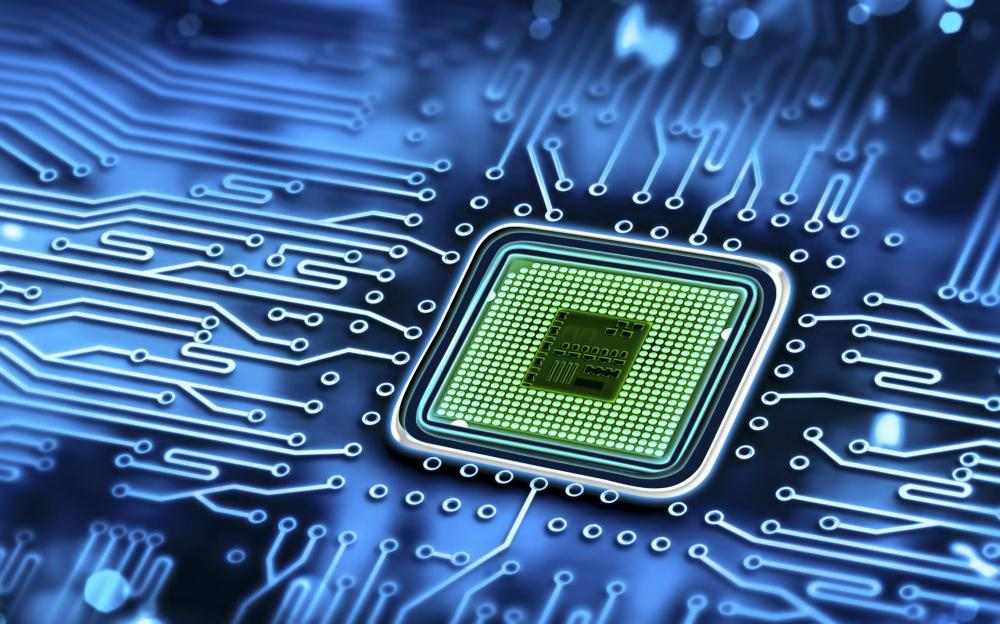# What Is a Digital Integrated Circuit?

Geisha A. Legazpi

A digital integrated circuit (IC) is a highly miniaturized electronic circuit, operating on individual voltage levels, fitted into a small package. It commonly operates with low-voltage direct current (DC) power supplies. Typical supply voltages are 5 and 3.3 volts (V). Earlier digital integrated circuits worked on 12 V power supplies.A simple digital integrated circuit can be seen in the form of a microchip.

Different types of semiconductors, resistors, and capacitors are used in the digital integrated circuit. The simplest digital integrated circuit could be a 14-pin dual-in-line package chip or microchip that has six built-in digital inverters. Each inverter uses a single pin for input and a single pin for output. The inverter outputs a “high” when a “low” is fed into the input, but when a “high” is fed into the input the output is “low.” This is why it is called an inverter.

In electronics, computers, cell phones, and digital devices, there are so many types of digital integrated circuits that perform all kinds of functions including mathematical calculations using the binary number system. In an 8-bit microcomputer, a set of 8 bits is referred to as a byte. Each byte can represent a number from 0 to 255, or it can represent –128 to +127. In certain modes of computation known as the binary-coded decimal, the byte can represent a number from 00 to 99.

There are analog integrated circuits that perform all sorts of analog functions, such as amplification, signal conversion, and detection. The digital integrated circuit, operating on digital levels, can distinguish the digital equivalent of analog signals by using a converter. The analog-to-digital (A/D) converter is a combined analog and digital circuit that inputs an analog level and outputs a multi-bit digital equivalent of the analog sample. For instance, an A/D converter may sample a 24 volts direct current (VDC) voltage and generate a byte with bit values “00011000,” which is “24.”

The digital-to-analog (D/A) converter is a more straightforward circuit that may not require an integrated circuit. By using passive devices, such as resistors and an analog amplifier, a D/A converter can be built. When space is minimal, a D/A converter chip may be a practical choice.

There are many circuits and equipment used in digital electronics. The microprocessor uses registers, which are sets of digital latches that retain a single bit. A 16-bit processor uses 16 of these latches that are capable of remembering only 1 bit each. For memory applications, the density of semiconductors in memory chips has increased to contain more than just a few tens of gigabytes per square inch. Each gigabyte is equivalent to a billion bytes, and each billion bytes is 8 billion bits.

## You might also Like